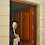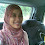## May 30, 2009

### Islamic Contribution to Mathematics

Mathematics

Muslim mathematicians excelled in geometry, as can be seen in their graphic arts, and it was the great Al-Biruni (who excelled also in the fields of natural history, even geology and mineralogy) who established trigonometry as a distinct branch of mathematics. Other Muslim mathematicians made significant progress in number theory.

It is interesting to note that Islam so strongly urges mankind to study and explore the universe. For example, the Noble Quran states:

"We (Allah) will show you (mankind) Our signs/patterns in the horizons/universe and in yourselves until you are convinced that the revelation is the truth."
[Noble Quran 41:53]
This invitation to explore and search made Muslims interested in astronomy, mathematics, chemistry, and the other sciences, and they had a very clear and firm understanding of the correspondences among geometry, mathematics, and astronomy.

The Muslims invented the symbol for zero (The word "cipher" comes from Arabic sifr), and they organized the numbers into the decimal system - base 10. Additionally, they invented the symbol to express an unknown quantity, i.e. variables like x.

The first great Muslim mathematician, Al-Khawarizmi, invented the subject of algebra (al-Jabr), which was further developed by others, most notably Umar Khayyam. Al-Khawarizmi's work, in Latin translation, brought the Arabic numerals along with the mathematics to Europe, through Spain. The word "algorithm" is derived from his name.

Calculus

Integral Calculus

Around 1000 AD, Al-Karaji, using mathematical induction, found a proof for the sum of integral cubes. The historian of mathematics, F. Woepcke, praised Al-Karaji for being "the first who introduced the theory of algebraic calculus." Shortly afterwards, Ibn al-Haytham (known as Alhazen in the West), an Iraqi mathematician working in Egypt, was the first mathematician to derive the formula for the sum of the fourth powers, and using an early proof by mathematical induction, he developed a method for determining the general formula for the sum of any integral powers. He used his result on sums of integral powers to perform an integration, in order to find the volume of a paraboloid. He was thus able to find the integrals for polynomials up to the fourth degree, and came close to finding a general formula for the integrals of any polynomials. This was fundamental to the development of infinitesimal and integral calculus. His results were repeated by the Moroccan mathematicians Abu-l-Hasan ibn Haydur (d. 1413) and Abu Abdallah ibn Ghazi (1437-1514), by Jamshīd al-Kāshī (c. 1380-1429) in The Calculator's Key, and by the Indian mathematicians of the Kerala school of astronomy and mathematics in the 15th-16th centuries.

Differential Calculus

In the 12th century, the Persian mathematician Sharaf al-Dīn al-Tūsī was the first to discover the derivative of cubic polynomials, an important result in differential calculus. His Treatise on Equations developed concepts related to differential calculus, such as the derivative function and the maxima and minima of curves, in order to solve cubic equations which may not have positive solutions. For example, in order to solve the equation$\ x^3 + a = bx$, al-Tusi finds the maximum point of the curve$\ bx - x^3 = a$. He uses the derivative of the function to find that the maximum point occurs at$x = \sqrt{\frac{b}{3}}$, and then finds the maximum value for y at$2(\frac{b}{3})^\frac{3}{2}$ by substituting$x = \sqrt{\frac{b}{3}}$ back into$\ y = bx - x^3$. He finds that the equation$\ bx - x^3 = a$ has a solution if$a \le 2(\frac{b}{3})^\frac{3}{2}$, and al-Tusi thus deduces that the equation has a positive root if$D = \frac{b^3}{27} - \frac{a^2}{4} \ge 0$, where D is the discriminant of the equation.

Sources

"Calculus." Wikipedia, the free encyclopedia. N.p., n.d. Web. 30 May 2009. .

"Mathematics." Science Islam - Proof From Truth. N.p., n.d. Web. 30 May 2009. .

1.Salam..
Ermm.. post that i've been waiting for..
congrat!

2.ok. i'll post more like this. thanks.

3.mentangmentang nak exam calculus!
haha.

all the best! wink*

4.ooh, menggunakan historical/bibliographical criticism